# NCERT solutions for Class 11 Physics chapter 7 - System of Particles and Rotational Motion [Latest edition]

#### Chapters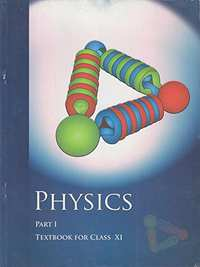## Solutions for Chapter 7: System of Particles and Rotational Motion

Below listed, you can find solutions for Chapter 7 of CBSE NCERT for Class 11 Physics.

Exercises
Exercises [Pages 178 - 181]

### NCERT solutions for Class 11 Physics Chapter 7 System of Particles and Rotational Motion Exercises [Pages 178 - 181]

Exercises | Q 1 | Page 178

Give the location of the centre of mass of a (i) sphere, (ii) cylinder, (iii) ring, and (iv) cube, each of uniform mass density. Does the centre of mass of a body necessarily lie inside the body?

Exercises | Q 2 | Page 178

In the HCl molecule, the separation between the nuclei of the two atoms is about 1.27 Å (1 Å = 10–10 m). Find the approximate location of the CM of the molecule, given that a chlorine atom is about 35.5 times as massive as a hydrogen atom and nearly all the mass of an atom is concentrated in its nucleus

Exercises | Q 3 | Page 178

A child sits stationary at one end of a long trolley moving uniformly with a speed Von a smooth horizontal floor. If the child gets up and runs about on the trolley in any manner, what is the speed of the CM of the (trolley + child) system?

Exercises | Q 5 | Page 178

Show that a. (b × c) is equal in magnitude to the volume of the parallelepiped formed on the three vectors, ab and c.

Exercises | Q 6 | Page 178

Find the components along the x, y, z axes of the angular momentum of a particle, whose position vector is with components xyand momentum is with components pxpy and 'p_z. Show that if the particle moves only in the x-plane the angular momentum has only a z-component.

Exercises | Q 7 | Page 178

Two particles, each of mass and speed v, travel in opposite directions along parallel lines separated by a distance d. Show that the vector angular momentum of the two particle system is the same whatever be the point about which the angular momentum is taken.

Exercises | Q 8 | Page 178

A non-uniform bar of weight is suspended at rest by two strings of negligible weight as shown in Fig.7.39. The angles made by the strings with the vertical are 36.9° and 53.1° respectively. The bar is 2 m long. Calculate the distance of the centre of gravity of the bar from its left end.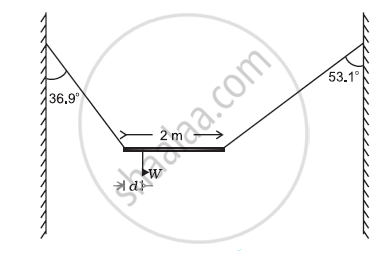Exercises | Q 9 | Page 178

A car weighs 1800 kg. The distance between its front and back axles is 1.8 m. Its centre of gravity is 1.05 m behind the front axle. Determine the force exerted by the level ground on each front wheel and each back wheel.

Exercises | Q 10.1 | Page 178

Given the moment of inertia of a disc of mass and radius about any of its diameters to be MR2/4, find its moment of inertia about an axis normal to the disc and passing through a point on its edge

Exercises | Q 10.1 | Page 178

Find the moment of inertia of a sphere about a tangent to the sphere, given the moment of inertia of the sphere about any of its diameters to be 2MR2/5, where is the mass of the sphere and is the radius of the sphere.

Exercises | Q 11 | Page 179

Torques of equal magnitude are applied to a hollow cylinder and a solid sphere, both having the same mass and radius. The cylinder is free to rotate about its standard axis of symmetry, and the sphere is free to rotate about an axis passing through its centre. Which of the two will acquire a greater angular speed after a given time?

Exercises | Q 12 | Page 179

A solid cylinder of mass 20 kg rotates about its axis with angular speed 100 rad s–1. The radius of the cylinder is 0.25 m. What is the kinetic energy associated with the rotation of the cylinder? What is the magnitude of the angular momentum of the cylinder about its axis?

Exercises | Q 13.1 | Page 179

Show that the child’s new kinetic energy of rotation is more than the initial kinetic energy of rotation. How do you account for this increase in kinetic energy?

Exercises | Q 13.1 | Page 179

A child stands at the centre of a turntable with his two arms outstretched. The turntable is set rotating with an angular speed of 40 rev/min. How much is the angular speed of the child if he folds his hands back and thereby reduces his moment of inertia to 2/5 times the initial value? Assume that the turntable rotates without friction.

Exercises | Q 14 | Page 179

A rope of negligible mass is wound round a hollow cylinder of mass 3 kg and radius 40 cm. What is the angular acceleration of the cylinder if the rope is pulled with a force of 30 N? What is the linear acceleration of the rope? Assume that there is no slipping.

Exercises | Q 15 | Page 179

To maintain a rotor at a uniform angular speed of 200 rad s–1, an engine needs to transmit a torque of 180 Nm. What is the power required by the engine?

(Note: uniform angular velocity in the absence of friction implies zero torque. In practice, applied torque is needed to counter frictional torque). Assume that the engine is 100 % efficient.

Exercises | Q 16 | Page 179

From a uniform disk of radius R, a circular hole of radius R/2 is cut out. The centre of the hole is at R/2 from the centre of the original disc. Locate the centre of gravity of the resulting flat body.

Exercises | Q 17 | Page 179

A metre stick is balanced on a knife edge at its centre. When two coins, each of mass 5 g are put one on top of the other at the 12.0 cm mark, the stick is found to be balanced at 45.0 cm. What is the mass of the metre stick?

Exercises | Q 18 | Page 179

A solid sphere rolls down two different inclined planes of the same heights but different angles of inclination. (a) Will it reach the bottom with the same speed in each case? (b) Will it take longer to roll down one plane than the other? (c) If so, which one and why?

Exercises | Q 19 | Page 179

A hoop of radius 2 m weighs 100 kg. It rolls along a horizontal floor so that its centre of mass has a speed of 20 cm/s. How much work has to be done to stop it?

Exercises | Q 20 | Page 179

The oxygen molecule has a mass of 5.30 × 10–26 kg and a moment of inertia of 1.94×10–46 kg m2 about an axis through its centre perpendicular to the lines joining the two atoms. Suppose the mean speed of such a molecule in a gas is 500 m/s and that its kinetic energy of rotation is two thirds of its kinetic energy of translation. Find the average angular velocity of the molecule.

Exercises | Q 21 | Page 179

A solid cylinder rolls up an inclined plane of angle of inclination 30°. At the bottom of the inclined plane, the centre of mass of the cylinder has a speed of 5 m/s.

(a) How far will the cylinder go up the plane?

Exercises | Q 22 | Page 179

As shown in Fig.7.40, the two sides of a step ladder BA and CA are 1.6 m long and hinged at A. A rope DE, 0.5 m is tied half way up. A weight 40 kg is suspended from a point F, 1.2 m from B along the ladder BA. Assuming the floor to be frictionless and neglecting the weight of the ladder, find the tension in the rope and forces exerted by the floor on the ladder. (Take = 9.8 m/s2)

(Hint: Consider the equilibrium of each side of the ladder separately.)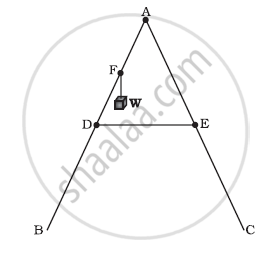Exercises | Q 23 | Page 180

A man stands on a rotating platform, with his arms stretched horizontally holding a 5 kg weight in each hand. The angular speed of the platform is 30 revolutions per minute. The man then brings his arms close to his body with the distance of each weight from the axis changing from 90cm to 20cm. The moment of inertia of the man together with the platform may be taken to be constant and equal to 7.6 kg m2.

(a) What is his new angular speed? (Neglect friction.)

(b) Is kinetic energy conserved in the process? If not, from where does the change come about?

Exercises | Q 24 | Page 180

A bullet of mass 10 g and speed 500 m/s is fired into a door and gets embedded exactly at the centre of the door. The door is 1.0 m wide and weighs 12 kg. It is hinged at one end and rotates about a vertical axis practically without friction. Find the angular speed of the door just after the bullet embeds into it.

(Hint: The moment of inertia of the door about the vertical axis at one end is ML2/3.)

Exercises | Q 25 | Page 180

Two discs of moments of inertia I1 and I2 about their respective axes (normal to the disc and passing through the centre), and rotating with angular speeds ω1 and ω2 are brought into contact face to face with their axes of rotation coincident. (a) What is the angular speed of the two-disc system? (b) Show that the kinetic energy of the combined system is less than the sum of the initial kinetic energies of the two discs. How do you account for this loss in energy? Take ω1 ≠ ω2.

Exercises | Q 26.1 | Page 180

Prove the theorem of perpendicular axes.

(Hint: Square of the distance of a point (x, y) in the x–y plane from an axis through the origin perpendicular to the plane is x+ y2).

Exercises | Q 26.2 | Page 180

Prove the theorem of parallel axes.

(Hint: If the centre of mass is chosen to be the origin summ_ir_i = 0

Exercises | Q 27 | Page 180

Prove the result that the velocity v of translation of a rolling body (like a ring, disc, cylinder or sphere) at the bottom of an inclined plane of a height h is given by v^2 = (2gh)/((1+k^2"/"R^2)).

Using dynamical consideration (i.e. by consideration of forces and torques). Note is the radius of gyration of the body about its symmetry axis, and R is the radius of the body. The body starts from rest at the top of the plane.

Exercises | Q 28 | Page 180

A disc rotating about its axis with angular speed ωois placed lightly (without any translational push) on a perfectly frictionless table. The radius of the disc is R. What are the linear velocities of the points A, B and C on the disc shown in Figure? Will the disc roll in the direction indicated?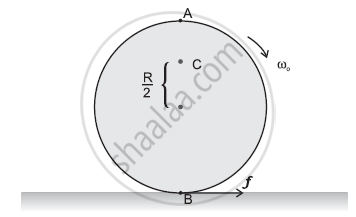Exercises | Q 29 | Page 181

Explain why friction is necessary to make the disc in Figure roll in the direction indicated

(a) Give the direction of frictional force at B, and the sense of frictional torque, before perfect rolling begins.

(b) What is the force of friction after perfect rolling begins?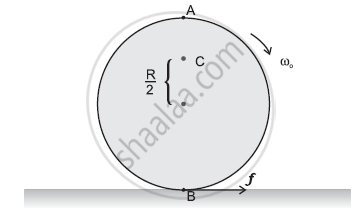Exercises | Q 30 | Page 181

A solid disc and a ring, both of radius 10 cm are placed on a horizontal table simultaneously, with initial angular speed equal to 10 π rad s-1. Which of the two will start to roll earlier? The coefficient of kinetic friction is μk = 0.2.

Exercises | Q 31 | Page 181

A cylinder of mass 10 kg and radius 15 cm is rolling perfectly on a plane of inclination 30°. The coefficient of static friction µs = 0.25.

(a) How much is the force of friction acting on the cylinder?

(b) What is the work done against friction during rolling?

(c) If the inclination θ of the plane is increased, at what value of θ does the cylinder begin to skid, and not roll perfectly?

Exercises | Q 32.1 | Page 181

Read each statement below carefully, and state, with reasons, if it is true or false;

During rolling, the force of friction acts in the same direction as the direction of motion of the CM of the body

Exercises | Q 32.2 | Page 181

Read each statement below carefully, and state, with reasons, if it is true or false;

The instantaneous speed of the point of contact during rolling is zero.

Exercises | Q 32.3 | Page 181

Read each statement below carefully, and state, with reasons, if it is true or false;

The instantaneous acceleration of the point of contact during rolling is zero.

Exercises | Q 32.4 | Page 181

Read each statement below carefully, and state, with reasons, if it is true or false;

For perfect rolling motion, work done against friction is zero.

Exercises | Q 32.5 | Page 181

Read each statement below carefully, and state, with reasons, if it is true or false;

A wheel moving down a perfectly frictionless inclined plane will undergo slipping (not rolling) motion

Exercises | Q 7.33 | Page 181

Separation of Motion of a system of particles into motion of the centre of mass and motion about the centre of mass:

1. Show pi = p’+ miV Where pi is the momentum of the ith particle (of mass mi)  and p′ i = mi v′ i. Note v′ i is the velocity of the ith particle relative to the centre of mass. Also, prove using the definition of the centre of mass sum"p""'"_"t" = 0
2. Show K = K′ + 1/2MV2

where K is the total kinetic energy of the system of particles, K′ is the total kinetic energy of the
system when the particle velocities are taken with respect to the centre of mass and MV2/2 is the
kinetic energy of the translation of the system as a whole (i.e. of the centre of mass motion of the
system). The result has been used in Sec. 7.14.

3. Show where "L""'" = sum"r""'"_"t" xx "p""'"_"t" is the angular momentum of the system about the centre of mass with
velocities taken relative to the centre of mass. Remember "r"_"t" = "r"_"t" - "R"; rest of the notation is the standard notation used in the chapter. Note L′ and MR × V can be said to be angular momenta, respectively, about and of the centre of mass of the system of particles.
4. Show "dL"^"'"/"dt" = ∑"r"_"i"^"'" xx "dP"^"'"/"dt"
Further show that "dL"^'/"dt" = τ_"ext"^"'"
Where "τ"_"ext"^"'"` is the sum of all external torques acting on the system about the centre of mass.
(Hint: Use the definition of centre of mass and third law of motion. Assume the internal forces between any two particles act along the line joining the particles.)

## Solutions for Chapter 7: System of Particles and Rotational Motion

Exercises## NCERT solutions for Class 11 Physics chapter 7 - System of Particles and Rotational Motion

Shaalaa.com has the CBSE Mathematics Class 11 Physics CBSE solutions in a manner that help students grasp basic concepts better and faster. The detailed, step-by-step solutions will help you understand the concepts better and clarify any confusion. NCERT solutions for Mathematics Class 11 Physics CBSE 7 (System of Particles and Rotational Motion) include all questions with answers and detailed explanations. This will clear students' doubts about questions and improve their application skills while preparing for board exams.

Further, we at Shaalaa.com provide such solutions so students can prepare for written exams. NCERT textbook solutions can be a core help for self-study and provide excellent self-help guidance for students.

Concepts covered in Class 11 Physics chapter 7 System of Particles and Rotational Motion are Dynamics of Rotational Motion About a Fixed Axis, Angular Momentum in Case of Rotation About a Fixed Axis, Rolling Motion, Momentum Conservation and Centre of Mass Motion, Centre of Mass of a Rigid Body, Centre of Mass of a Uniform Rod, Rigid Body Rotation, Equilibrium of Rigid Body, Moment of Inertia, Theorems of Perpendicular and Parallel Axes, Kinematics of Rotational Motion About a Fixed Axis, Motion - Rigid Body, Motion of Centre of Mass, Linear Momentum of a System of Particles, Vector Product of Two Vectors, Angular Velocity and Its Relation with Linear Velocity, Torque and Angular Momentum, Centre of Mass, Equations of Rotational Motion, Comparison of Linear and Rotational Motions, Values of Moments of Inertia for Simple Geometrical Objects (No Derivation).

Using NCERT Class 11 Physics solutions System of Particles and Rotational Motion exercise by students is an easy way to prepare for the exams, as they involve solutions arranged chapter-wise and also page-wise. The questions involved in NCERT Solutions are essential questions that can be asked in the final exam. Maximum CBSE Class 11 Physics students prefer NCERT Textbook Solutions to score more in exams.

Get the free view of Chapter 7, System of Particles and Rotational Motion Class 11 Physics additional questions for Mathematics Class 11 Physics CBSE, and you can use Shaalaa.com to keep it handy for your exam preparation.

Share